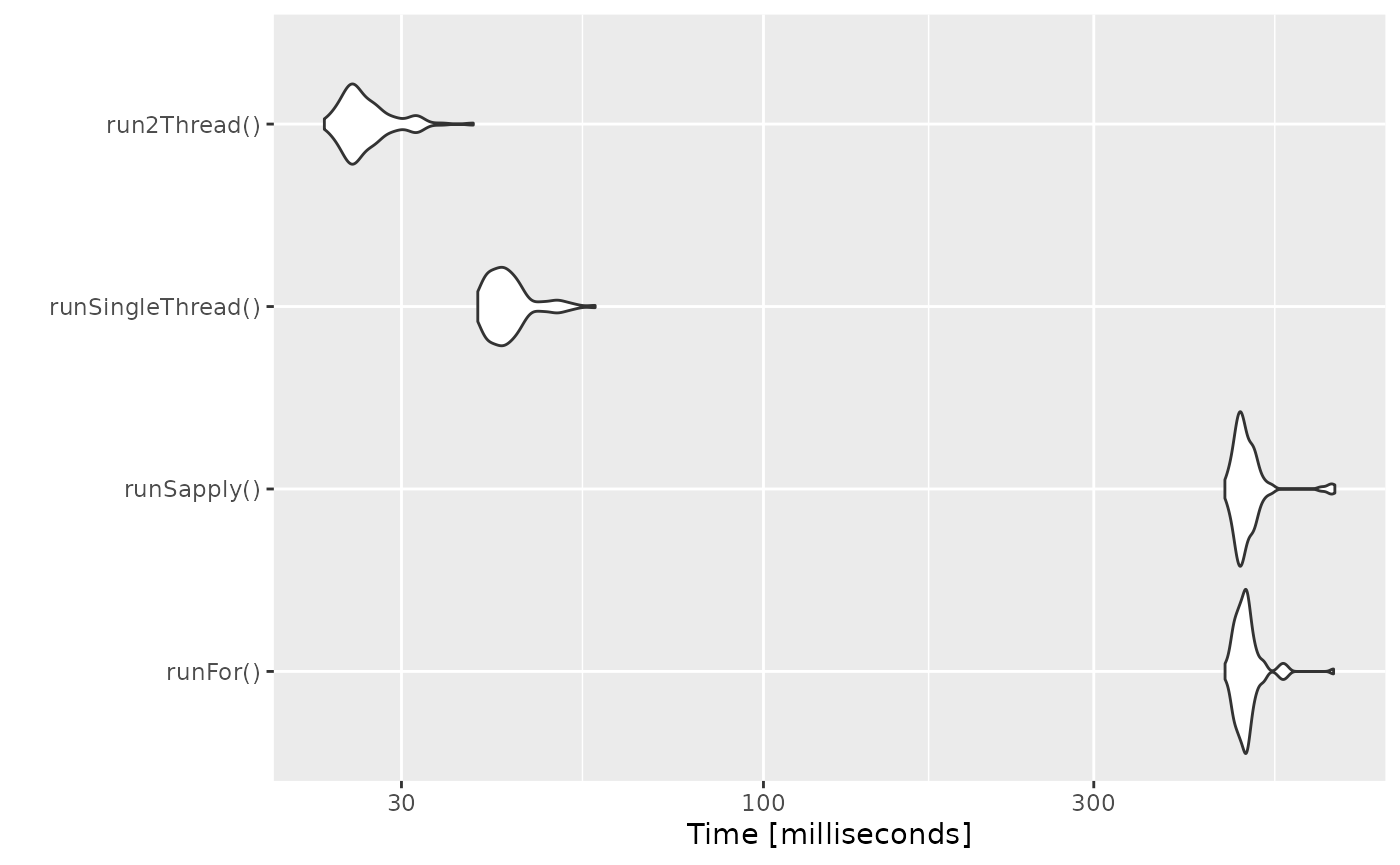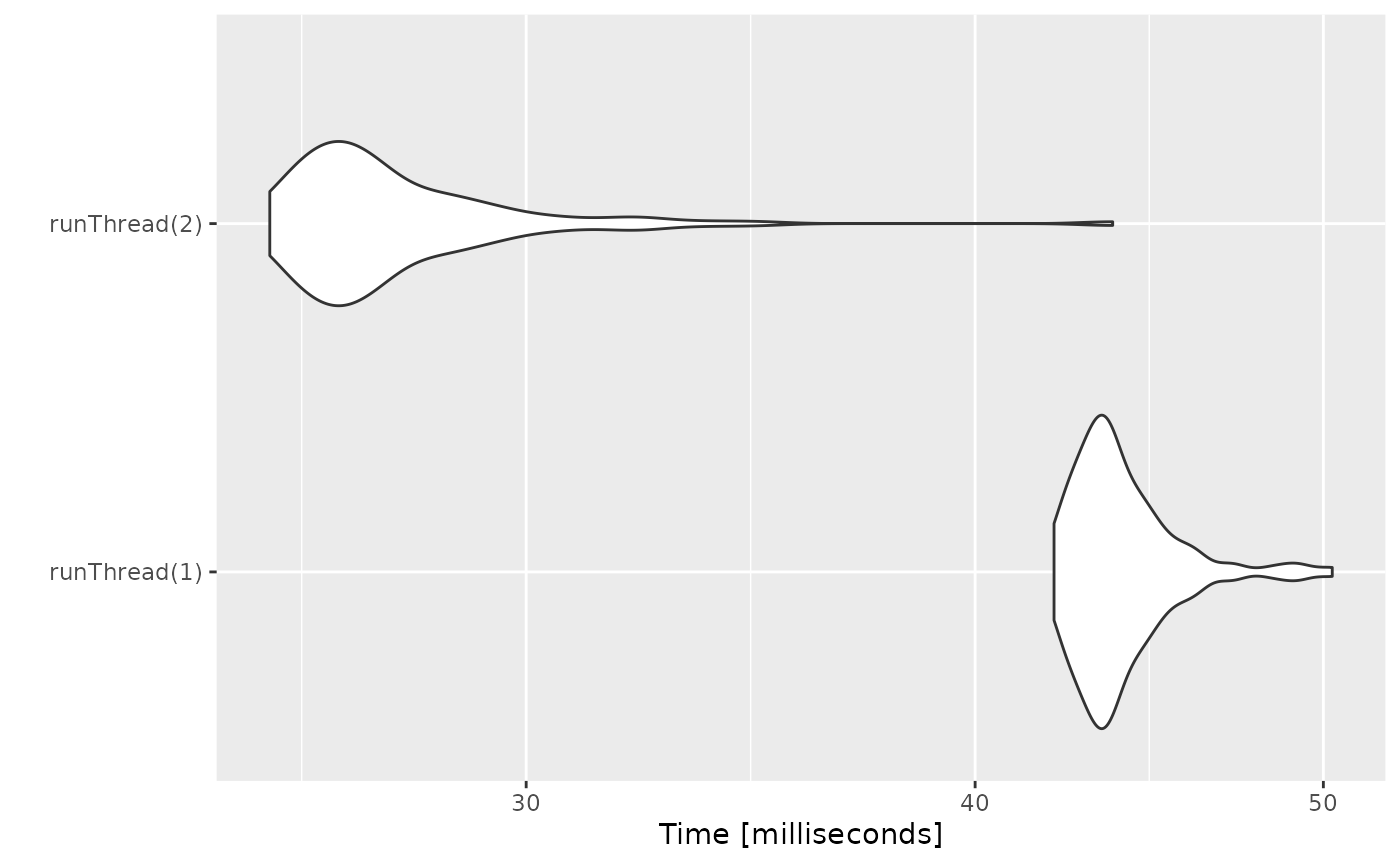## Increasing rxode2 speed by multi-subject parallel solving

rxode2 originally developed as an ODE solver that allowed an ODE solve for a single subject. This flexibility is still supported.

The original code from the rxode2 tutorial is below:


library(rxode2)
#>   no cache: create with rxCreateCache()

library(microbenchmark)
library(ggplot2)

mod1 <- rxode2({
C2 = centr/V2;
C3 = peri/V3;
d/dt(depot) = -KA*depot;
d/dt(centr) = KA*depot - CL*C2 - Q*C2 + Q*C3;
d/dt(peri) = Q*C2 - Q*C3;
d/dt(eff) = Kin - Kout*(1-C2/(EC50+C2))*eff;
eff(0) = 1
})
#> using C compiler: ‘gcc (Ubuntu 11.4.0-1ubuntu1~22.04) 11.4.0’

## Create an event table

ev <- et() %>%

nsub <- 100 # 100 sub-problems
sigma <- matrix(c(0.09,0.08,0.08,0.25),2,2) # IIV covariance matrix
mv <- rxRmvn(n=nsub, rep(0,2), sigma) # Sample from covariance matrix
CL <- 7*exp(mv[,1])
V2 <- 40*exp(mv[,2])
params.all <- cbind(KA=0.3, CL=CL, V2=V2, Q=10, V3=300,
Kin=0.2, Kout=0.2, EC50=8)

### For Loop

The slowest way to code this is to use a for loop. In this example we will enclose it in a function to compare timing.

runFor <- function(){
res <- NULL
for (i in 1:nsub) {
params <- params.all[i,]
x <- mod1$solve(params, ev) ##Store results for effect compartment res <- cbind(res, x[, "eff"]) } return(res) } ### Running with apply In general for R, the apply types of functions perform better than a for loop, so the tutorial also suggests this speed enhancement runSapply <- function(){ res <- apply(params.all, 1, function(theta) mod1$run(theta, ev)[, "eff"])
}

### Run using a single-threaded solve

You can also have rxode2 solve all the subject simultaneously without collecting the results in R, using a single threaded solve.

The data output is slightly different here, but still gives the same information:

runSingleThread <- function(){
solve(mod1, params.all, ev, cores=1)[,c("sim.id", "time", "eff")]
}

### Run a 2 threaded solve

rxode2 supports multi-threaded solves, so another option is to have 2 threads (called cores in the solve options, you can see the options in rxControl() or rxSolve()).

run2Thread <- function(){
solve(mod1, params.all, ev, cores=2)[,c("sim.id", "time", "eff")]
}

### Compare the times between all the methods

Now the moment of truth, the timings:

bench <- microbenchmark(runFor(), runSapply(), runSingleThread(),run2Thread())
print(bench)
#> Unit: milliseconds
#>               expr      min        lq      mean   median       uq      max
#>           runFor() 464.2462 485.17780 499.43750 496.4963 504.0652 666.0799
#>        runSapply() 464.0028 485.23760 501.54662 493.5743 508.9063 668.8894
#>  runSingleThread()  38.6705  40.53545  42.96348  42.0920  44.0078  57.1383
#>       run2Thread()  23.2149  25.14385  26.74644  25.9965  27.5635  38.1001
#>  neval
#>    100
#>    100
#>    100
#>    100
autoplot(bench)It is clear that the largest jump in performance when using the solve method and providing all the parameters to rxode2 to solve without looping over each subject with either a for or a sapply. The number of cores/threads applied to the solve also plays a role in the solving.

We can explore the number of threads further with the following code:

runThread <- function(n){
solve(mod1, params.all, ev, cores=n)[,c("sim.id", "time", "eff")]
}

bench <- eval(parse(text=sprintf("microbenchmark(%s)",
collapse=","))))
print(bench)
#> Unit: milliseconds
#>          expr     min       lq     mean  median       uq     max neval
#>  runThread(1) 42.0746 42.99965 43.95447 43.5344 44.44740 50.2861   100
#>  runThread(2) 25.4550 26.33460 27.66737 26.9168 28.39075 43.6866   100
autoplot(bench)There can be a suite spot in speed vs number or cores. The system type (mac, linux, windows and/or processor), complexity of the ODE solving and the number of subjects may affect this arbitrary number of threads. 4 threads is a good number to use without any prior knowledge because most systems these days have at least 4 threads (or 2 processors with 4 threads).

## A real life example

Before some of the parallel solving was implemented, the fastest way to run rxode2 was with lapply. This is how Rik Schoemaker created the data-set for nlmixr comparisons, but reduced to run faster automatic building of the pkgdown website.

library(rxode2)
library(data.table)
#Define the rxode2 model
ode1 <- "
d/dt(abs)    = -KA*abs;
d/dt(centr)  =  KA*abs-(CL/V)*centr;
C2=centr/V;
"

#Create the rxode2 simulation object
mod1 <- rxode2(model = ode1)
#> using C compiler: ‘gcc (Ubuntu 11.4.0-1ubuntu1~22.04) 11.4.0’

#Population parameter values on log-scale
paramsl <- c(CL = log(4),
V = log(70),
KA = log(1))
#make 10,000 subjects to sample from:
nsubg <- 300 # subjects per dose
doses <- c(10, 30, 60, 120)
nsub <- nsubg * length(doses)
#IIV of 30% for each parameter
omega <- diag(c(0.09, 0.09, 0.09))# IIV covariance matrix
sigma <- 0.2
#Sample from the multivariate normal
set.seed(98176247)
rxSetSeed(98176247)
library(MASS)
mv <-
mvrnorm(nsub, rep(0, dim(omega)), omega) # Sample from covariance matrix
#Combine population parameters with IIV
params.all <-
data.table(
"ID" = seq(1:nsub),
"CL" = exp(paramsl['CL'] + mv[, 1]),
"V" = exp(paramsl['V'] + mv[, 2]),
"KA" = exp(paramsl['KA'] + mv[, 3])
)
#set the doses (looping through the 4 doses)
params.all[, AMT := rep(100 * doses,nsubg)]

Startlapply <- Sys.time()

#Run the simulations using lapply for speed
s = lapply(1:nsub, function(i) {
#selects the parameters associated with the subject to be simulated
params <- params.all[i]
#creates an eventTable with 7 doses every 24 hours
ev <- eventTable()
ev$add.dosing( dose = params$AMT,
nbr.doses = 1,
dosing.to = 1,
rate = NULL,
start.time = 0
)
#generates 4 random samples in a 24 hour period
ev$add.sampling(c(0, sort(round(sample(runif(600, 0, 1440), 4) / 60, 2)))) #runs the rxode2 simulation x <- as.data.table(mod1$run(params, ev))
#merges the parameters and ID number to the simulation output
x[, names(params) := params]
})

#runs the entire sequence of 100 subjects and binds the results to the object res
res = as.data.table(do.call("rbind", s))

Stoplapply <- Sys.time()

print(Stoplapply - Startlapply)
#> Time difference of 23.8137 secs

By applying some of the new parallel solving concepts you can simply run the same simulation both with less code and faster:

rx <- rxode2({
CL =  log(4)
V = log(70)
KA = log(1)
CL = exp(CL + eta.CL)
V = exp(V + eta.V)
KA = exp(KA + eta.KA)
d/dt(abs)    = -KA*abs;
d/dt(centr)  =  KA*abs-(CL/V)*centr;
C2=centr/V;
})
#> using C compiler: ‘gcc (Ubuntu 11.4.0-1ubuntu1~22.04) 11.4.0’

omega <- lotri(eta.CL ~ 0.09,
eta.V ~ 0.09,
eta.KA ~ 0.09)

doses <- c(10, 30, 60, 120)

startParallel <- Sys.time()
ev <- do.call("rbind",
lapply(seq_along(doses), function(i){
et() %>%
et(amt=doses[i]) %>% # Add single dose
et(0) %>% # Add 0 observation
## Generate 4 samples in 24 hour period
et(lapply(1:4, function(...){c(0, 24)})) %>%
et(id=seq(1, nsubg) + (i - 1) * nsubg) %>%
## Convert to data frame to skip sorting the data
## When binding the data together
as.data.frame
}))
## To better compare, use the same output, that is data.table
res <- rxSolve(rx, ev, omega=omega, returnType="data.table")
endParallel <- Sys.time()
print(endParallel - startParallel)
#> Time difference of 0.2003043 secs

You can see a striking time difference between the two methods; A few things to keep in mind:

• rxode2 use the thread-safe sitmo threefry routines for simulation of eta values. Therefore the results are expected to be different (also the random samples are taken in a different order which would be different)

• This prior simulation was run in R 3.5, which has a different random number generator so the results in this simulation will be different from the actual nlmixr comparison when using the slower simulation.

• This speed comparison used data.table. rxode2 uses data.table internally (when available) try to speed up sorting, so this would be different than installations where data.table is not installed. You can force rxode2 to use order() when sorting by using forderForceBase(TRUE). In this case there is little difference between the two, though in other examples data.table’s presence leads to a speed increase (and less likely it could lead to a slowdown).

### Want more ways to run multi-subject simulations

The version since the tutorial has even more ways to run multi-subject simulations, including adding variability in sampling and dosing times with et() (see rxode2 events for more information), ability to supply both an omega and sigma matrix as well as adding as a thetaMat to R to simulate with uncertainty in the omega, sigma and theta matrices; see rxode2 simulation vignette.

### Session Information

The session information:

sessionInfo()
#> R version 4.3.1 (2023-06-16)
#> Platform: x86_64-pc-linux-gnu (64-bit)
#> Running under: Ubuntu 22.04.3 LTS
#>
#> Matrix products: default
#> LAPACK: /usr/lib/x86_64-linux-gnu/openblas-pthread/libopenblasp-r0.3.20.so;  LAPACK version 3.10.0
#>
#> locale:
#>   LC_CTYPE=C.UTF-8       LC_NUMERIC=C           LC_TIME=C.UTF-8
#>   LC_COLLATE=C.UTF-8     LC_MONETARY=C.UTF-8    LC_MESSAGES=C.UTF-8
#>  LC_TELEPHONE=C         LC_MEASUREMENT=C.UTF-8 LC_IDENTIFICATION=C
#>
#> time zone: UTC
#> tzcode source: system (glibc)
#>
#> attached base packages:
#>  stats     graphics  grDevices utils     datasets  methods   base
#>
#> other attached packages:
#>  MASS_7.3-60           data.table_1.14.8     ggplot2_3.4.3
#>  microbenchmark_1.4.10 rxode2_2.0.13.9000
#>
#> loaded via a namespace (and not attached):
#>   sass_0.4.7               utf8_1.2.3               generics_0.1.3
#>   lattice_0.21-8           stringi_1.7.12           digest_0.6.33
#>   magrittr_2.0.3           evaluate_0.21            grid_4.3.1
#>  rxode2random_2.0.11.9001 fastmap_1.1.1            lotri_0.4.3
#>  rprojroot_2.0.3          jsonlite_1.8.7           rxode2ll_2.0.11.9000
#>  backports_1.4.1          purrr_1.0.2              fansi_1.0.4
#>  scales_1.2.1             textshaping_0.3.6        jquerylib_0.1.4
#>  RApiSerialize_0.1.2      cli_3.6.1                crayon_1.5.2
#>  rlang_1.1.1              units_0.8-3              munsell_0.5.0
#>  withr_2.5.0              cachem_1.0.8             yaml_2.3.7
#>  rxode2et_2.0.10.9000     tools_4.3.1              qs_0.25.5
#>  rxode2parse_2.0.16.9000  memoise_2.0.1            checkmate_2.2.0
#>  dplyr_1.1.2              colorspace_2.1-0         vctrs_0.6.3
#>  R6_2.5.1                 lifecycle_1.0.3          stringr_1.5.0
#>  fs_1.6.3                 stringfish_0.15.8        ragg_1.2.5
#>  PreciseSums_0.6          pkgconfig_2.0.3          desc_1.4.2
#>  RcppParallel_5.1.7       pkgdown_2.0.7            bslib_0.5.1
#>  pillar_1.9.0             gtable_0.3.3             glue_1.6.2
#>  Rcpp_1.0.11              systemfonts_1.0.4        highr_0.10
#>  xfun_0.40                tibble_3.2.1             tidyselect_1.2.0
#>  sys_3.4.2                knitr_1.43               farver_2.1.1
#>  dparser_1.3.1-10         nlme_3.1-162             htmltools_0.5.6
#>  rmarkdown_2.24           compiler_4.3.1